# RF sheet resistance in multilayer media

Click here to go to our main page on RF sheet resistance

Along with this discussion we offer a download that calculates equivalent RF sheet resistance for metals that have up to three different layers. If you are a thin film vendor and would like to sponsor this outstanding page and beat your competitors to the punch, let us know!

By the way, we use the metric system for this discussion, and in the download. Why? because there are no popular units of bulk resistivity in the English system. Regarding skin depths and thin film thicknesses, if you want to convert from microns to micro-inches exactly, divide microns by 0.0254. There are approximately 40 microinches in one micron, that's close enough when you are dealing with plating.

Here is a clickable index for this web page:

Solving the skin depth equation for multiple layers

Some useful examples of RF sheet resistance calculations

Nickel plating

Thin-film TaN resistors

### Solving the skin depth equation for multiple layers

Quite often we use a stack of multiple metals when constructing a thin film or a MMIC. To reduce processing cost, resistor material is often placed underneath the primary conductor metal(s), such as gold. How do you analyze this?

Look at the image below... here, delta is skin depth of the particular material, and sigma is its conductivity.  The Y axis is normalized in thickness divided by skin depths.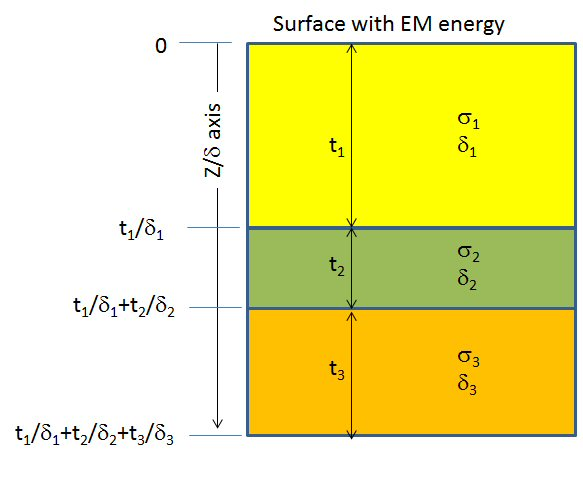The conductivity of the overall metal structure when exposed to a signal on the top surface is solved using calculus.  We will omit how the solution was set up here, but it pays to remember at least some small part of what you learned in college.  Below are equations for sheet conductivity (the reciprocal of sheet resistance), starting with one metal (note: the equations below were corrected slightly on April 11, 2016, sorry for any inconvenience...)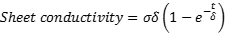Now two metals: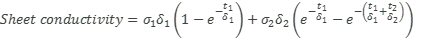Now three metals: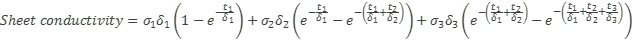See the pattern? See how we "integrate" the conductivity across the X-axis where that axis is in skin depths, not microns?

Below is the normal current density versus skin depth curve, which you should have memorized. Someone spelled "number" wrong....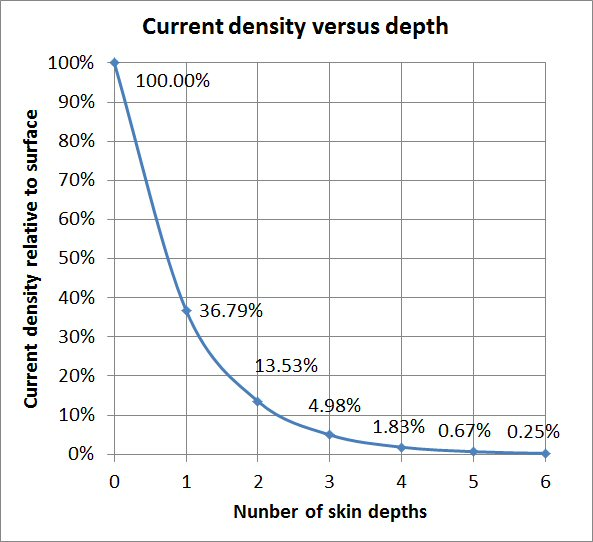Now check out what happens in a two metal system, at three frequencies: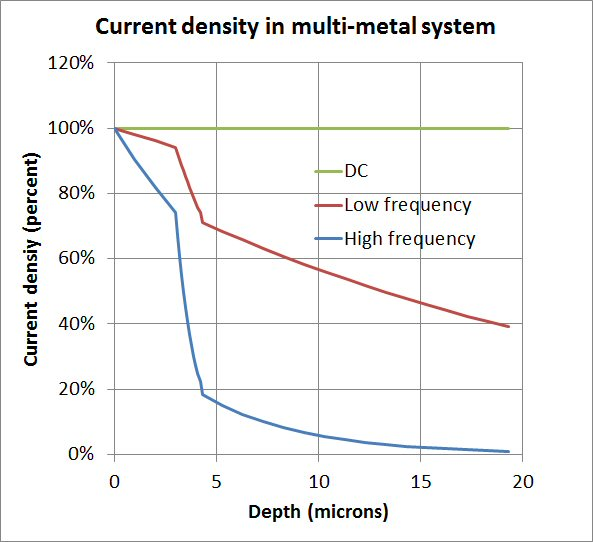Come back in a few days, we'll annotate this a lot better!

More to come!

### Some examples

Below are some examples of data generated by the Microwaves101 RF sheet resistance calculator download.

#### Nickel plating

New for November 2015: this warning came from Reto:

If you include strongly ferro-magnetic materials any results past a few GHz or so will not be realistic. Permeability is a strong function of frequency once you go past a few GHz. The magnetic domains can’t follow the fast changing magnetic field anymore and relative permeability drops down towards unity. Nickel, for example, with an initial relative permeability of 100 -600, will drop to somewhere between 1 and 2 at 10 GHz. Permeability and frequency are both in the denominator of the skin-depth formula. In the frequency range where the relative permeability drops from several hundred to about 1, the frequency only increases 10-fold. So the skin depth is actually increasing in that frequency range. Hence the sheet resistance (assuming that layer is much thicker than one skin depth) is actually dropping in that range of frequency.

Let's look at the effect of 50 micro-inches of nickel plating. As you can see from the plot below, from 1 GHz on up you have over five skin depths. So you have achieved the "maximum RF sheet conductivity" of the metal at any microwave frequency.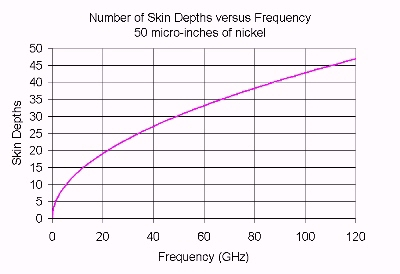Below is what that means in terms of RF sheet resistance. A "good" value for RF sheet resistance is perhaps 0.03 ohms per square at X-band. Here we have about 1 ohm per square at X-band. If your transmission lines have any length at all, you have some serious attenuation in a fifty-ohm system. Note that if you over plate the nickel with gold, you don't fix the problem.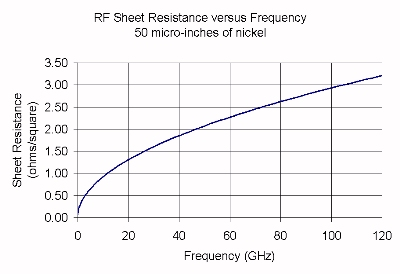#### Thin-film TaN resistors

Let's look at the RF sheet resistance of two thickness of tantalum nitride (TaN) resistors. In the first case, the Tan film to achieve a DC sheet resistance of 50 ohms per square is about is 2 microinches, or 0.05 microns. This sheet resistance is a popular value with thin film vendors. This thickness of Tan is less than 1% of a skin depth at X-band, so the RF sheet resistance is very nearly equal to the DC value. The plot below shows how the RF skin depth varies over frequency; the error is only about 1% all the way up at W-band, less at lower frequencies. In this case there is almost nothing to concern yourself with.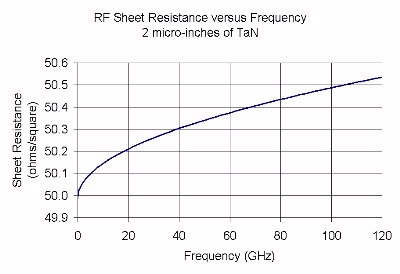Below we have simulated a thicker film of TaN, this time approximately 20 micro-inches, which provides a DC sheet resistance of 5 ohms per square. In this case the film is about 6% of a skin depth a X-band, and about 20% at W-band. Below the RF sheet resistance is plotted over frequency. Now we see a 3% error at X-band and a 10% error at W-band. Something to think about next time you are trying to trying to design an accurate attenuator at 100 GHz!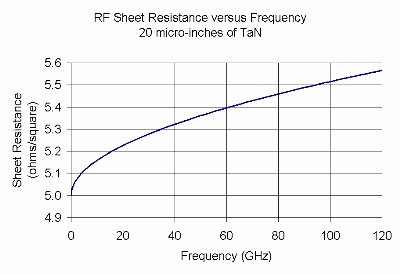### Overplating resistor material

In the case of a thin film that has a resistor layer below the gold, does the resistor metal have an appreciable effect on the transmission line attenuation? Here you want the resistor material to be a fraction of a skin depth (a DC value of 50 ohms/square is good), while the gold to be at least three skin depths. Then you won't be able to tell the difference between a gold plated over Tan, or pure gold!

Author : Unknown Editor